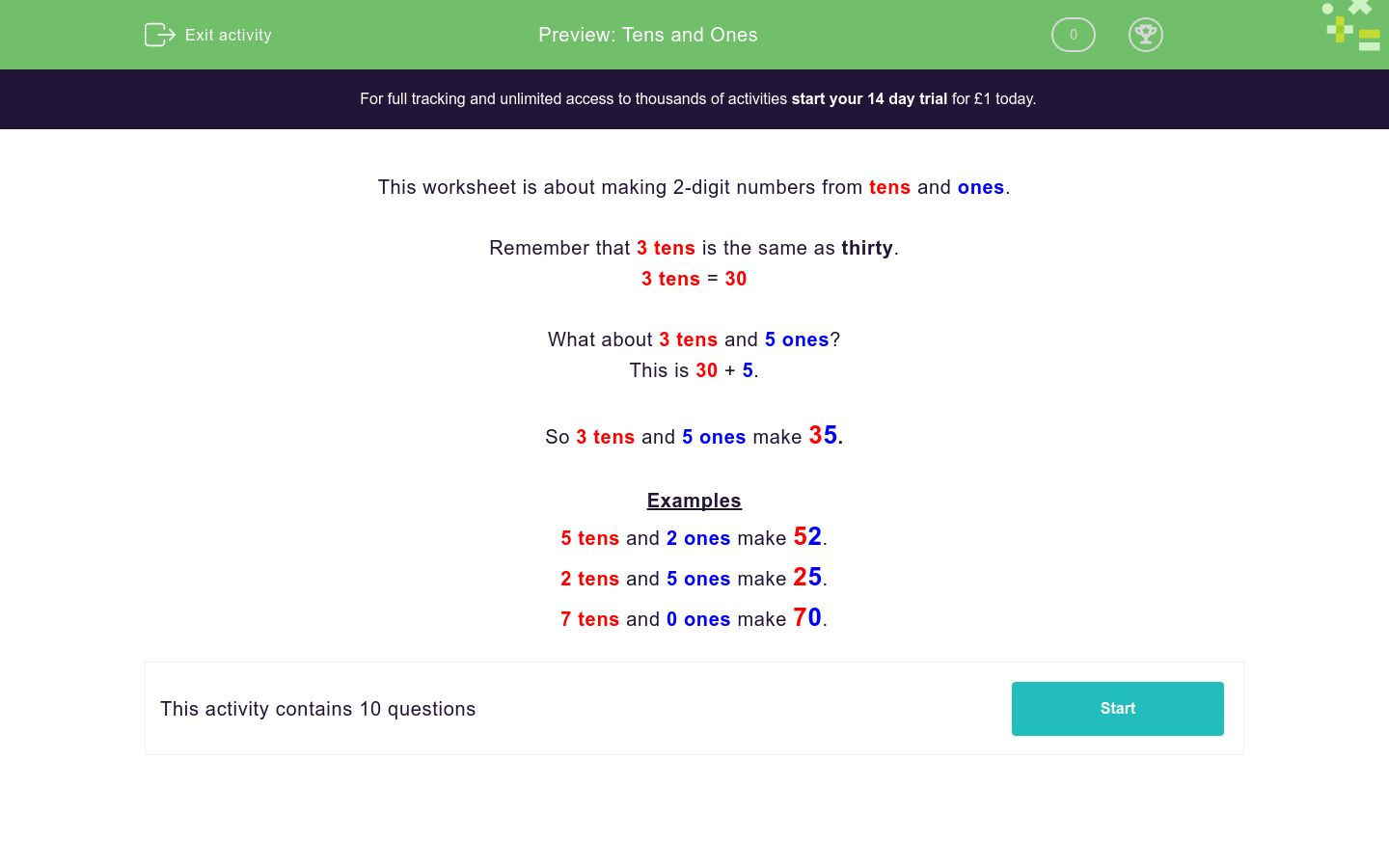# Tens and Ones

In this worksheet, students change tens and ones to make 2-digit numbers.Key stage:  KS 1

Curriculum topic:   Number: Number and Place Value

Curriculum subtopic:   Recognise Tens and Ones

Difficulty level:### QUESTION 1 of 10

This worksheet is about making 2-digit numbers from tens and ones.

Remember that 3 tens is the same as thirty.

3 tens = 30

What about 3 tens and 5 ones?

This is 30 + 5.

So 3 tens and 5 ones make 35.

Examples

5 tens and 2 ones make 52.

2 tens and 5 ones make 25.

7 tens and 0 ones make 70.

Make a 2-digit number from:

3 tens and 1 one

Make a 2-digit number from:

9 tens and 6 ones

Make a 2-digit number from:

2 tens and 2 ones

Make a 2-digit number from:

5 tens and 0 ones

Make a 2-digit number from:

5 tens and 3 ones

Make a 2-digit number from:

4 tens and 6 ones

Make a 2-digit number from:

2 tens and 3 ones

Make a 2-digit number from:

7 tens and 5 ones

Make a 2-digit number from:

4 tens and 8 ones

Make a 2-digit number from:

6 tens and 9 ones

• Question 1

Make a 2-digit number from:

3 tens and 1 one

31
EDDIE SAYS
30 + 1
• Question 2

Make a 2-digit number from:

9 tens and 6 ones

96
EDDIE SAYS
90 + 6
• Question 3

Make a 2-digit number from:

2 tens and 2 ones

22
EDDIE SAYS
20 + 2
• Question 4

Make a 2-digit number from:

5 tens and 0 ones

50
EDDIE SAYS
50 + 0
• Question 5

Make a 2-digit number from:

5 tens and 3 ones

53
EDDIE SAYS
50 + 3
• Question 6

Make a 2-digit number from:

4 tens and 6 ones

46
EDDIE SAYS
40 + 6
• Question 7

Make a 2-digit number from:

2 tens and 3 ones

23
EDDIE SAYS
20 + 3
• Question 8

Make a 2-digit number from:

7 tens and 5 ones

75
EDDIE SAYS
70 + 5
• Question 9

Make a 2-digit number from:

4 tens and 8 ones

48
EDDIE SAYS
40 + 8
• Question 10

Make a 2-digit number from:

6 tens and 9 ones

69
EDDIE SAYS
60 + 9
---- OR ----

Sign up for a £1 trial so you can track and measure your child's progress on this activity.

### What is EdPlace?

We're your National Curriculum aligned online education content provider helping each child succeed in English, maths and science from year 1 to GCSE. With an EdPlace account you’ll be able to track and measure progress, helping each child achieve their best. We build confidence and attainment by personalising each child’s learning at a level that suits them.

Get started